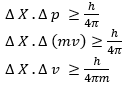# Heisenberg Uncertainity Principle (chemistry)

## Heisenberg Uncertainty Principle

According to this, it is impossible to determine simultaneously the exact position & the exact momentum (or velocity) of an electron
Mathematically:• Δx is uncertainty in position Δ v is in velocity.
• If the position of the electron is known with high degree of accuracy ($\Delta x$ is small), then the velocity of the electron will be uncertain [$\Delta (v_x)$ is large]. On the other hand, if the velocity of the electron is known precisely ($\Delta (v_x )$ is small), then the position of the electron will be uncertain ($\Delta x$ will be large).
• So, if we carry out some physical measurements on the electron's position or velocity, the outcome will always depict a fuzzy or blur picture
• The effect of this principle is only significant for microscopic objects and it is negligibile for macroscopic objects
Question 1
If the position of electron is measured with accuracy of 0.002 nm. Calculate uncertainty in momentum of e
Solution
$\Delta x. \Delta p = \frac {h}{4 \pi}$
$0.002 \times 10^(-9 ).\Delta p= \frac {6.6 \times 10^{-34}}{4 \times 3.14}$
$\Delta p= \frac {6.6 \times 10^{-34}}{4 \times 3.14 \times 0.002 \times 10^{-9} }$
= 2.637 X 10-23 kg m/s

Question 2
A microscope using suitable photons is employed to locate an electron in an atom within a distance of 0.1 A0. What is the uncertainty involved in the measurement of its velocity?
Solution
$\Delta x. \Delta p = \frac {h}{4 \pi}$
or
$\Delta x. m \Delta v = \frac {h}{4 \pi}$
$\Delta v= \frac {h}{4 m \pi \Delta x}$
Now $\Delta x= .1 A^0 = .1 \times 10^{-10}$ m
Substituting all the values
$\Delta v = \frac {6.626 \times 10^{-34}}{4 \times 3.14 \times .1 \times 10^{-10} \times 9.11 \times 10^{-31}}$
$=5.79 \times 10^6$ m/s

Question 3
A golf ball has a mass of 40g, and a speed of 45 m/s. If the speed can be measured within accuracy of 2%, calculate the uncertainty in the position. ?
Solution
The uncertainty in the speed is 2%, i.e.
$\Delta v= 45 \times \frac {2}{100} = .9$ m/s
Now
$\Delta x= \frac {h}{4 m \pi \Delta v}$
Substituting all the values, we get
$\Delta x=1.46 \times 10^{-33}$ m

Question 4
If uncertainty in position of electron is zero, the uncertainty in its momentum would be
(i)zero
(ii) $> \frac {h}{4 \pi}$
(iii) $< \frac {h}{2 \pi}$
(iv)infinity
Solution
(iv)

Question 5
if uncertainty in position and momentum are equal then uncertainty in velocity is
(i)$\sqrt {\frac {h}{2 \pi}}$
(ii) $\frac {1}{2m} \sqrt {\frac {h}{\pi}}$
(iii)$\sqrt {\frac {h}{ \pi}}$
(iv)None
Solution
$\Delta x. \Delta p = \frac {h}{4 \pi}$
The uncertainty in position and momentum are equal.let it be y,then
$y^2 = \frac {h}{4 \pi}$
$y= \sqrt {\frac {h}{4 \pi}}$
Now
$m \Delta v = \sqrt {\frac {h}{4 \pi}}$
or
$\Delta v= \frac {1}{2m} \sqrt {\frac {h}{\pi}}$

### Significance of Uncertainty Principle:-

1. It rules out definite paths or orbits of electrons and other similar particles.
2. This principle is significant only for microscope object like electrons and negligible for macroscopic objects

### Reason for failure of Bohr model:-

1. The wave character is not considered in Bohr model.
2. According to Bohr model, an orbit is clearly defined path & this path can completely be defined only if both position & velocity of electron are known as same lime. This is not possible according to Heisenberg uncertainty principle.